# Texas Go Math Grade 4 Unit 6 Answer Key Personal Financial Literacy

Refer to our Texas Go Math Grade 4 Answer Key Pdf to score good marks in the exams. Test yourself by practicing the problems from Texas Go Math Grade 4 Unit 6 Answer Key Personal Financial Literacy.

## Texas Go Math Grade 4 Unit 6 Answer Key Personal Financial Literacy

Show What You Know

Add Decimals Estimate. Then find the sum.

Question 1.
Estimate:Question 2.
Estimate: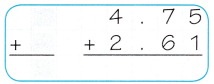Subtract Decimals Estimate. Then find the difference.

Question 3.
Estimate: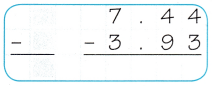Question 4.
Estimate:Multiply 3-Digit and 4-Digit Numbers by 1-Digit Numbers

Find the product.

Question 5.
684 × 7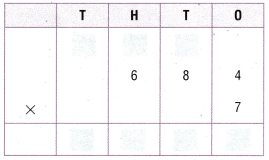Question 6.
1,157 × 4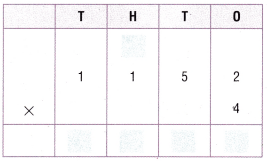Vocabulary Builder

Preview Wards

• budget
• financial institution
• fixed expense
• interest
• loan
• profit
• variable expense

Visualize It

Write an example for each term in the chart.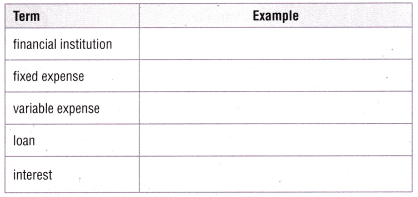Understand Vocabulary

Complete the sentences by using the preview words.

Question 1.
An organized plan for spending and saving money is called a __________.

Question 2.
A __________ is one in which the amount changes based on need or choice.

Question 3.
A __________ occurs regularly and the amount does not change.

Question 4.
Money that is lent by a bank or other financial institution is called a __________ .

Question 5.
__________ is the amount left after all the expenses are subtracted from the amount of money received from selling an item or service.

Question 6.
A __________ keeps money safe, lends money, and borrows money.

Vocabulary

You can use what you know about adding and subtracting whole numbers to add and subtract money amounts.

1. On the way home from school, Janine bought a bunch of bananas for $3.00. She gave the clerk a ten-dollar bill. How much change should she get back?Answer: An input/output table shows how input numbers and output numbers are related. A rule tells what to do to the input to get the output. You can use an input/output table to help you solve problems. Janine wants to know how much it will cost her to buy 4 bunches of bananas. Complete the input/output table to show how much the bananas will cost.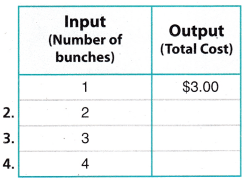5. The rule is: ______________ 6. Four bunches of bananas will cost ______________ . 7. How much would 8 bunches of bananas cost? ______________ Answer: Writing Make an input/output table that shows the total cost of 5 DVDs if each DVD costs$13.00.

Reading Look for this book in your library. Once Upon a Dime: A Math Adventure, by Nancy Kelly Allen

Make a Profit

Object of the Game Determine expenses and calculate the profit.

Materials

• 3 number cubes each labeled 1-6
• Monthly Worksheet
• Pencil or marker

Set Up

Each player becomes the owner of a made up company that sells items. Each player uses a worksheet to find the company’s expenses and profit for one month.

Number of Players: 2 to 4 players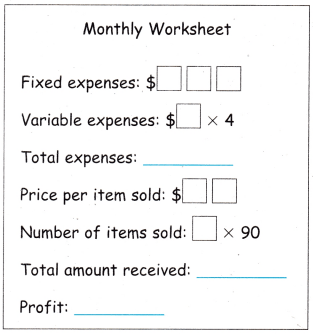How to Play

Question 1.
Player 1 tosses 3 cubes and arranges the digits in any order to determine the company’s fixed expenses.

Question 2.
Player 1 tosses one cube, and multiple by 4 to determine the company’s monthly variable expenses. Player 1 then finds their total expenses.

Question 3.
Player 1 tosses two cubes and arranges the digits in any order to determine the price of each item sold.

Question 4.
Player 1 tosses one cube and multiplies by 90 to determine the number of items sold. Player 1 then finds the total amount received for the number of items sold.